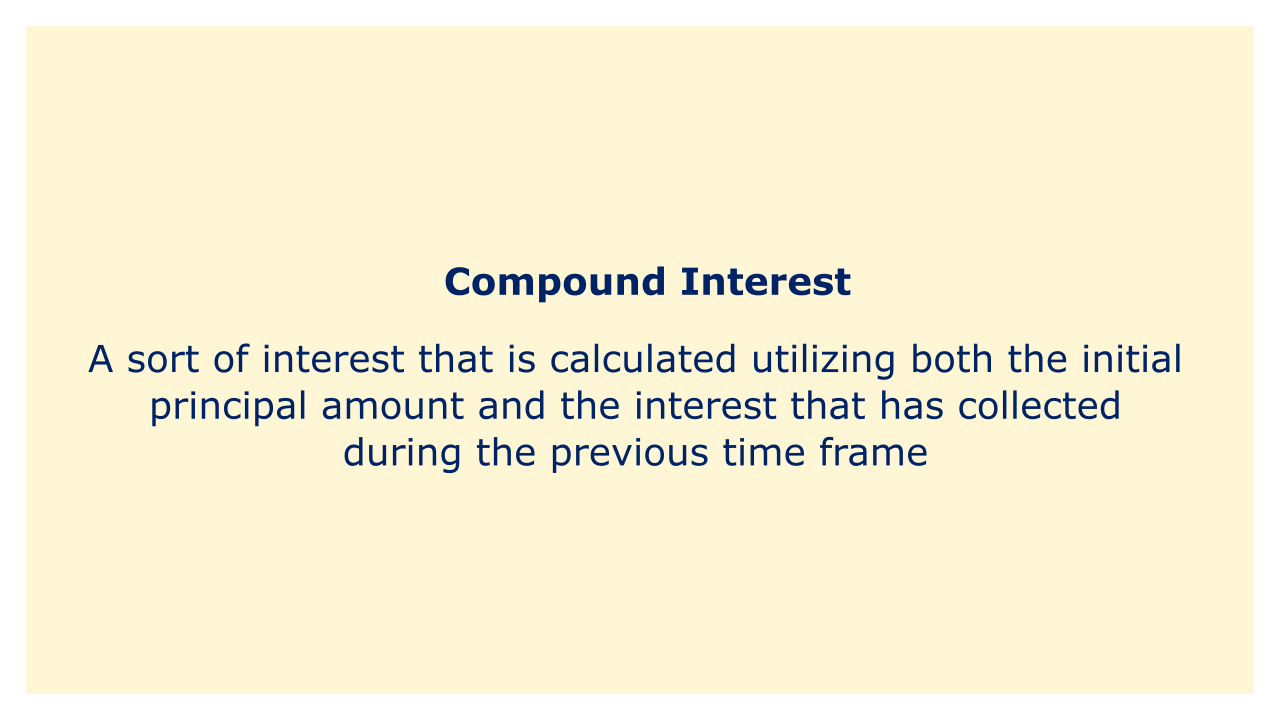# Compound InterestImage: Moneybestpal.com

### A type of interest called compound interest is calculated using both the starting principle sum and the interest that has accrued over the prior time period. It differs from basic interest, which only considers the starting principle amount and ignores interest from prior periods. Compound interest is commonly abbreviated C.I. in mathematics.

Compound interest is calculated by using the following formula:

C.I = P (1 + r/n)^(nt) - P

where P is the principal amount, r is the annual interest rate, n is the number of times interest is compounded per year, and t is the number of years.

For example, if a loan of \$10,000 is borrowed at an annual interest rate of 10%, compounded monthly, for 5 years, the compound interest of the loan is:

C.I = \$10,000 (1 + 0.1/12)^(12 x 5) - \$10,000

C.I = \$10,000 (1.0083)^(60) - \$10,000

C.I = \$16,470.09 - \$10,000

C.I = \$6,470.09

Compound interest, commonly referred to as the "power of compound interest," creates "interest on interest" and enables the principal amount to increase exponentially over time. In the long run, compound interest can boost returns and wealth for investors and savers, so it can be advantageous for them. The long-term growth in costs and obligations brought on by compound interest can be harmful to both debtors and borrowers.

The frequency schedule for compound interest can be set to occur annually, semiannually, quarterly, monthly, daily, or constantly. Since interest is applied to the principal amount more frequently the higher the frequency of compounding, the bigger the compound interest and total amount. Compound interest accrues at a higher rate when interest is added to the principal, hence the lesser the frequency of compounding, the smaller the final sum and compound interest. By changing the values of r and n, it is possible to adapt the compound interest formula to take into account various compounding frequencies.

Using a compound interest calculator, which is a tool for estimating compound interest and the total cost of an investment or a loan gave the values of P, r, n, and t, one can calculate compound interest. The effects of various compounding frequencies, interest rates, or time periods on the compound interest and the total amount can be compared using a compound interest calculator.
Tags